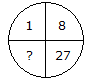# Verbal Reasoning - Character Puzzles - Discussion

### Discussion :: Character Puzzles - Character Puzzles 1 (Q.No.10)

10.

Which one will replace the question mark ?[A]. 41 [B]. 64 [C]. 35 [D]. 61

Explanation:

(1)3 = 1, (2)3 = 8, (3)3 = 27

Therefore, (4)3 = 64.

 Saikumart said: (Mar 20, 2016) Cube = 1^3 = 1; 2^3 = 8; 3^3 = 27; 4^3 = 64.

 Saikumart said: (Mar 20, 2016) Cube = 1^3 = 1; 2^3 = 8; 3^3 = 27; 4^3 = 64.

 Lucky said: (Feb 23, 2017) According to me, Consider the given circle as 100. i.e 1+8+27=36. So, 100 - 36 = 64.

 Krishna said: (May 13, 2019) That circular observation was astonishing. Thanks.

 Rashmi Ranjan said: (Nov 5, 2020) 1^3=1 2^3=8 3^3=27 Therefore (4)3 = 64.

 Tanuja Narasimha said: (Sep 28, 2021) Nice. Very interesting. Thanks for explaining.